## 哈弗H6受欢迎的背后：我们需要什么样的车

2019年03月30日 14:36 来源：车行天下 超过：9880次关注

环境改变带动需求变化，放眼当下的汽车市场，过去轿车“一家独大”的局面已经不复存在，SUV后来居上，已经成为了当下最热门的车型。近期的销量报告上，可以看到SUV的市场占比越来越大，几乎占领了销量市场的半壁江山。而在SUV的销量排行榜中，哈弗H6累计蝉联了69个月的销量冠军，可谓是自主品牌的骄傲，更是成为了人们心中的“国民神车”。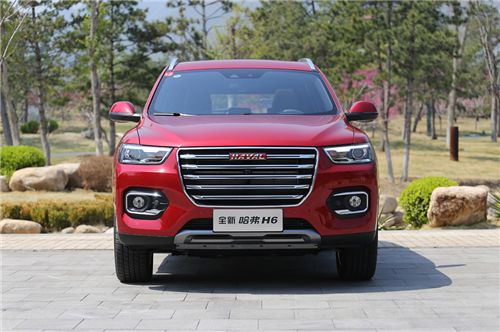为什么像全新哈弗H6这样的SUV车型会以如此迅猛的速度发展起来，哪些优势让人们对其情有独钟呢？

一、出行安全

全新哈弗H6在车身选材方面是很有良心的，其采用包骨络环状车身架构、65%高强度钢激光焊接车身，获得C-NCAP五星评定，在公开挑战翻滚实验中以优异表现通过严苛测试。同时，相比于同级别的轿车来说，SUV的空间更大，即便是意外发生时，可溃缩的空间也要比轿车多，能更好的保证车内乘客的安全。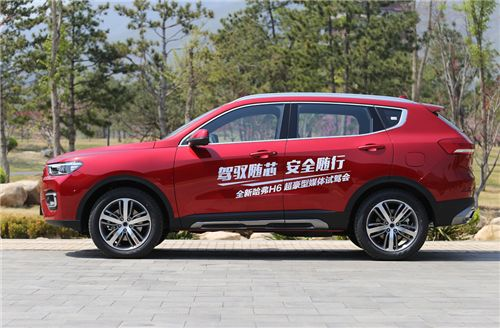而我们的销量冠军——全新哈弗H6不仅在设计上进行了创新，更是在安全配置上下足了功夫，配备奥托立夫主副驾驶座气囊、前排侧气囊、侧气帘共6个安全气囊，为驾乘人员带来全方位的安全保护，可以说，如此优秀的安全表现让开车的人有信心，也让家人更安心。

二、驾驶舒适度

我们在来说SUV的第二个优势，就是空间大，舒适度高。坐过SUV和轿车的人都知道，轿车的空间较为狭小且局限性大，在座位上的坐姿也比较矮，长时间驾驶或乘坐，容易出现疲劳感。不过，这个问题在全新哈弗H6这样的SUV上迎刃而解。出色的空间不会给乘坐带来压抑感，同时全新哈弗H6也更重视细节上的处理，进一步加大乘坐舒适度，其座椅包裹性很好，软硬程度适中，不会觉得长途驾驶时座椅对身体的支持性不够。要知道全新哈弗H6的长宽高分别为4600/1860/1720毫米，轴距为2680毫米，在主要的市场竞争对手面前，它的优势还是十分明显的，无论前排还是后排的乘客，都可以获得一个不错的乘坐体验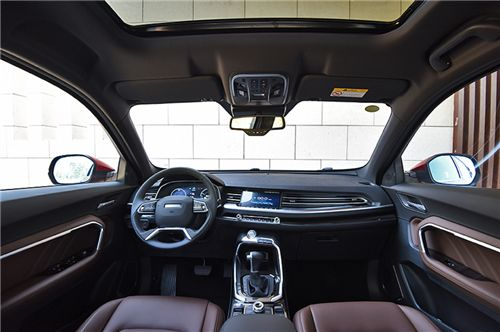三、澎湃动力

无论对于轿车或者是SUV，想要在驾驶体验上得到进一步的提升，动力的强弱就是决定的关键。全新哈弗H6所搭载的1.5GDIT发动机动力充沛,最大功率为124kW、峰值扭矩为285Nm，路上想超车时,也只需轻点油门就能轻松完成超越。另外,这款发动机还配备有CVVL技术和智能启停技术，配合由长城汽车自主研发的七速湿式双离合变速器，让大家获得更好的驾驶体验，又同时带来非常可观的油耗表现，从而进一步降低你的出行成本。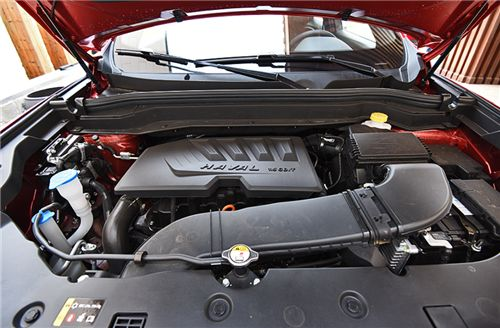全新哈弗H6不仅动力表现充沛，而且对车内噪音的控制也做的十分出色。以往我所乘坐的紧凑级SUV中，都会有后排噪音大过于前排的现象。不过哈弗H6的前后排噪音差别不大，即便是坐在后排也不会被滋扰到，而且座椅靠背角度和座垫长短非常合适，175cm的我坐在后排时的空间非常充裕。

总结：

对于全新哈弗H6这样的“国民神车”来说，它之所以备受消费者青睐，并且累计69个月持续霸占销量榜头椅，绝不仅仅因为它是一款实用性强的家用SUV，在这些光辉“战绩”和名誉背后，，更是与哈弗品牌的高性价比定位和其自身全面而出色的产品表现有着密不可分的关系。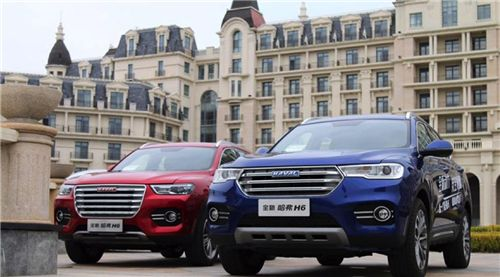现在全新哈弗H6已经是人们心中的“国民神车”，出色的实力表现也让其在市场中的位置更加的稳固。相信哈弗也会持续在技术上加大研发力度，推陈出新，让产品竞争力越来越强，真真正正让消费者受益！

#### 相关文章

0-500 字已有评论 0条 查看评论>>

### 热门标签

﻿
• 快速找车
• 选择品牌
• 选择品牌
• A  奥迪
• A  阿斯顿·马丁
• A  阿尔法·罗密欧
• B  宝沃
• B  布加迪
• B  巴博斯
• B  保时捷
• B  宾利
• B  奔驰
• B  宝马
• B  本田
• B  别克
• B  标致
• B  比亚迪
• B  宝骏
• B  北汽制造
• B  北汽新能源
• B  北汽幻速
• B  北汽威旺
• B  北京汽车
• B  奔腾
• B  北汽绅宝
• C  长安
• C  长安商用
• C  长城
• C  昌河
• D  大众
• D  道奇
• D  DS
• D  东南
• D  东风风神
• D  东风风行
• D  东风小康
• D  东风风度
• D  东风
• F  福特
• F  丰田
• F  菲亚特
• F  法拉利
• F  福田
• F  福迪
• F  福汽启腾
• G  观致
• G  广汽传祺
• G  广汽吉奥
• G  GMC
• H  红旗
• H  汉腾汽车
• H  哈弗
• H  哈飞
• H  海格
• H  海马
• H  华颂
• H  黄海
• H  华泰
• H  恒天
• J  吉利汽车
• J  捷豹
• J  Jeep
• J  江淮
• J  江铃
• J  金杯
• J  九龙
• J  金旅
• K  凯翼
• K  凯迪拉克
• K  克莱斯勒
• K  科尼塞克
• K  卡威
• K  开瑞
• L  路虎
• L  林肯
• L  劳斯莱斯
• L  兰博基尼
• L  雷克萨斯
• L  铃木
• L  雷诺
• L  理念
• L  力帆
• L  莲花汽车
• L  猎豹
• L  路特斯
• L  陆风
• M  马自达
• M  MG
• M  MINI
• M  玛莎拉蒂
• M  摩根
• M  迈凯轮
• N  纳智捷
• O  欧宝
• O  讴歌
• O  欧朗
• Q  奇瑞
• Q  起亚
• Q  启辰
• R  日产
• R  荣威
• R  瑞麒
• S  三菱
• S  斯威汽车
• S  萨博
• S  smart
• S  斯柯达
• S  斯巴鲁
• S  思铭
• S  双龙
• S  上汽大通
• S  双环
• T  特斯拉
• T  腾势
• W  沃尔沃
• W  五菱汽车
• W  五十铃
• W  威兹曼
• W  威麟
• X  现代
• X  雪佛兰
• X  雪铁龙
• X  西雅特
• Y  一汽
• Y  英菲尼迪
• Y  英致
• Y  依维柯
• Y  野马汽车
• Y  永源
• Z  众泰
• Z  中华
• Z  中兴
• Z  知豆
• 选择车系
• 选择车系
• 车型对比
• 选择品牌
• 选择品牌
• A  奥迪
• A  阿斯顿·马丁
• A  阿尔法·罗密欧
• B  宝沃
• B  布加迪
• B  巴博斯
• B  保时捷
• B  宾利
• B  奔驰
• B  宝马
• B  本田
• B  别克
• B  标致
• B  比亚迪
• B  宝骏
• B  北汽制造
• B  北汽新能源
• B  北汽幻速
• B  北汽威旺
• B  北京汽车
• B  奔腾
• B  北汽绅宝
• C  长安
• C  长安商用
• C  长城
• C  昌河
• D  大众
• D  道奇
• D  DS
• D  东南
• D  东风风神
• D  东风风行
• D  东风小康
• D  东风风度
• D  东风
• F  福特
• F  丰田
• F  菲亚特
• F  法拉利
• F  福田
• F  福迪
• F  福汽启腾
• G  观致
• G  广汽传祺
• G  广汽吉奥
• G  GMC
• H  红旗
• H  汉腾汽车
• H  哈弗
• H  哈飞
• H  海格
• H  海马
• H  华颂
• H  黄海
• H  华泰
• H  恒天
• J  吉利汽车
• J  捷豹
• J  Jeep
• J  江淮
• J  江铃
• J  金杯
• J  九龙
• J  金旅
• K  凯翼
• K  凯迪拉克
• K  克莱斯勒
• K  科尼塞克
• K  卡威
• K  开瑞
• L  路虎
• L  林肯
• L  劳斯莱斯
• L  兰博基尼
• L  雷克萨斯
• L  铃木
• L  雷诺
• L  理念
• L  力帆
• L  莲花汽车
• L  猎豹
• L  路特斯
• L  陆风
• M  马自达
• M  MG
• M  MINI
• M  玛莎拉蒂
• M  摩根
• M  迈凯轮
• N  纳智捷
• O  欧宝
• O  讴歌
• O  欧朗
• Q  奇瑞
• Q  起亚
• Q  启辰
• R  日产
• R  荣威
• R  瑞麒
• S  三菱
• S  斯威汽车
• S  萨博
• S  smart
• S  斯柯达
• S  斯巴鲁
• S  思铭
• S  双龙
• S  上汽大通
• S  双环
• T  特斯拉
• T  腾势
• W  沃尔沃
• W  五菱汽车
• W  五十铃
• W  威兹曼
• W  威麟
• X  现代
• X  雪佛兰
• X  雪铁龙
• X  西雅特
• Y  一汽
• Y  英菲尼迪
• Y  英致
• Y  依维柯
• Y  野马汽车
• Y  永源
• Z  众泰
• Z  中华
• Z  中兴
• Z  知豆
• 选择车系
• 选择车系
• 选择车型
• 选择车型
• 意见反馈